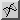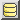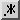HomeMemory managementJava equivalent to...constnewdeletemalloc# Unsigned arithmetic in Java

In C/C++, when you declare a variable as unsigned, the compiler will know to perform unsigned arithmetic on that variable. Arithmetic operations are operations such as addition and division, where we're not simply treating the number as a "raw string of bits", but where the bits have some "numerical" meaning. Perhaps less obviously, arithmetic operations include comparison. In our look at the Java equivalent of unsigned, we mentioned that bitwise operations— such as EOR, AND etc, where we do just treat the number as a series of bits— are essentially the same whether or not we later treat the resulting integer as signed or unsigned. So here, we just look at the tricky case of arithmetic operations.

Performing unsigned arithmetic in Java can be tricky, because in most cases, Java only "natively" caters for signed arithmetic: that is, it expects to interpret the top bit of an integer type (int, but also byte, short and long) as the sign bit.

## 32-bit unsigned arithmetic (and below)

If you are dealing purely with numbers that are 32 bits or smaller— i.e. the size of a Java int— then you can perform unsigned arithmetic reliably by using a long to perofmr the arithmetic. Only the top bit of a long is interpreted as the sign bit. So provided you keep to the bottom bits, you will effectively be performing unsigned arithmetic. We just need to be careful of two things:

• To cast a signed int to an unsigned value in the bottom 32 bits of a long, we need to AND with 0xffffffffL (232-1— or the number with all 32 bottom bits set).
• After an operation on longs that we're treating as unsigned ints, we should either cast back to an int, or and with 0xffffffffL if we want to keep the (unsigned) value in a long.

Wherever the result of an operation could be larger than the bottom 32 bits, we "chop off" the top 32 bits of the long. We can chop them off by casting back to an int, or ANDing with 0xffffffffL if we want to keep the result as a long, e.g. for further arithmetic. For example, here is one way to perform an unsigned 32-bit division in Java:

```public static int unsignedDiv(int i1, int i2) {
long l1 = i1 & 0xffffffffL, l2 = i2 & 0xffffffffL;
return (int) (l1 / l2);
}
```

We can perform an unsigned 32-bit comparison by "casting" the numbers to a long (remembering to AND with the bottom 32 bits as mentioned), then perform the comparison on the longs:

```public static boolean lessThanUnsigned(int n1, int n2) {
return (n1 & 0xffffffffL) < (n2 & 0xffffffffL);
}
```

## 64-bit unsigned arithmetic

Performing 64-bit unsigned arithmetic in Java is a bit more tricky, because there's no "next size up" primitive that you can use. We need to watch out for "special cases" and "manuall" interpret the sign bit as magnitude. In general:

• unsigned addition, subtraction and multiplication are identical: we can just use the ordinary +, - and * operators;
• unsigned comparison and unsigned division need special code.

The idea of unsigned addition/subtraction being identical to their signed counterparts may seem counterintuitive, but numbers are deliberately stored in a form (called "two's complement"), that allows them to be identical at the bit level.

### 64-bit unsigned comparison

We're of course talking about "less than" or "greater than": "equals" or "not equals" are of course identical whether the number is signed or unsigned. We'll take the example of unsigned less than. To understand how the comparison works, we start by considering the four possible combinations of the sign bit set or not set on the two numbers (henceforth x and y). In signed land, if the sign bit is set, this would mean less than zero. But we want to re-interpret that as is a big number— or more specifically, is greater or equal to 1<<31. So our "truth table" of sign bit set or not set for the two numbers looks as follows:

"Truth table" for an unsigned comparison x < y using signed arithmetic
Top bit of x
Top bit of y01
0x < y
(Signed comparison)
false
1truex < y
(Signed comparison)

The idea is that if the sign bits are the same, then we can perform an "ordinary" signed comparison— in effect the result will be the same as just comparing the bottom 63 bits. If the sign bits are different, then the number that has the sign bit set (is really "negative" as a signed number) should be treated as bigger, and vice versa. In other words, if the sign bits are different, we reverse the comparison. Logically this would look as follows:

```public static boolean isLessThanUnsigned(long n1, long n2) {
boolean comp = (n1 < n2);
if ((n1 < 0) != (n2 < 0)) {
comp = !comp;
}
return comp;
}
```

However, with a bit of bitwise trickery, we can actually write this as a single expression. It's not very common in Java, but we can use the exclusive OR operator (^) with booleans. The result of x ^ y is:

• x, if y is 0;
• opposite of x, if y is 1.

This means we can write the above as:

```public static boolean isLessThanUnsigned(long n1, long n2) {
return (n1 < n2) ^ ((n1 < 0) != (n2 < 0));
}
```

Since this code looks fairly obscure if you haven't had it explained, I would recommend always putting it inside a well-named method such as this. The method should be simple enough for most JIT compilers to inline, so there shouldn't be a performance hit.